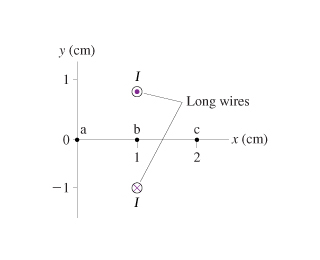# Problem: I = 8.0A(a) What is the magnetic field B at point a in the figure? Give your answer as vector.(b) What is the magnetic field B at point b in the figure? Give your answer as vector.(c) What is the magnetic field B at point c in the figure? Give your answer as vector.

###### FREE Expert Solution

Magnetic field due to a current-carrying conductor,

$\overline{){\mathbit{B}}{\mathbf{=}}\frac{{\mathbf{\mu }}_{\mathbf{0}}\mathbf{I}}{\mathbf{2}\mathbf{\pi r}}}$

Let the top conductor be 1 and lower conductor 2.

Vector magnitude,

$\overline{){\mathbf{r}}{\mathbf{=}}\sqrt{{\mathbf{x}}^{\mathbf{2}}\mathbf{+}{\mathbf{y}}^{\mathbf{2}}}}$

$\overline{){{\mathbf{r}}}_{\mathbf{x}\mathbf{,}\mathbf{y}}{\mathbf{=}}{\mathbf{\left(}}{{\mathbf{x}}}_{{\mathbf{2}}}{\mathbf{-}}{{\mathbf{x}}}_{{\mathbf{1}}}{\mathbf{\right)}}{\mathbf{,}}{\mathbf{\left(}}{{\mathbf{y}}}_{{\mathbf{2}}}{\mathbf{-}}{{\mathbf{y}}}_{{\mathbf{1}}}{\mathbf{\right)}}}$

Direction,

$\overline{){\mathbf{tan}}{\mathbit{\theta }}{\mathbf{=}}\frac{{\mathbit{A}}_{\mathbit{y}}}{{\mathbit{A}}_{\mathbit{x}}}}$

Resolving components,

Part A

${\mathbit{r}}_{\mathbf{1}\mathbf{,}\mathbf{a}}\mathbf{=}\sqrt{{\mathbf{\left(}\mathbf{0}\mathbf{.}\mathbf{01}\mathbf{-}\mathbf{0}\mathbf{\right)}}^{\mathbf{2}}\mathbf{+}{\mathbf{\left(}\mathbf{0}\mathbf{.}\mathbf{01}\mathbf{-}\mathbf{0}\mathbf{\right)}}^{\mathbf{2}}}$

r1,a = 0.01414 m

Direction,

$\mathbit{\theta }\mathbf{=}\mathbit{t}\mathbit{a}{\mathbit{n}}^{\mathbf{-}\mathbf{1}}\mathbf{\left(}\frac{\mathbf{0}\mathbf{.}\mathbf{01}}{\mathbf{0}\mathbf{.}\mathbf{01}}\mathbf{\right)}$

θ = 45°

${\mathbit{r}}_{\mathbf{2}\mathbf{,}\mathbf{a}}\mathbf{=}\sqrt{{\mathbf{\left(}\mathbf{0}\mathbf{.}\mathbf{01}\mathbf{-}\mathbf{0}\mathbf{\right)}}^{\mathbf{2}}\mathbf{+}{\mathbf{\left(}\mathbf{-}\mathbf{0}\mathbf{.}\mathbf{01}\mathbf{-}\mathbf{0}\mathbf{\right)}}^{\mathbf{2}}}$

r2,a = 0.01414 m

Direction,

$\mathbit{\theta }\mathbf{=}\mathbit{t}\mathbit{a}{\mathbit{n}}^{\mathbf{-}\mathbf{1}}\mathbf{\left(}\frac{\mathbf{-}\mathbf{0}\mathbf{.}\mathbf{01}}{\mathbf{0}\mathbf{.}\mathbf{01}}\mathbf{\right)}$

θ = - 45°

I = 8.0A

μ0 = 4π × 10−7 N/A2

${\mathbit{B}}_{\mathbf{1}\mathbf{,}\mathbf{a}}\mathbf{=}\frac{\mathbf{\left(}\mathbf{4}\mathbf{\pi }\mathbf{×}{\mathbf{10}}^{\mathbf{-}\mathbf{7}}\mathbf{\right)}\mathbf{8}\mathbf{.}\mathbf{0}}{\mathbf{2}\mathbf{\pi }\mathbf{×}\mathbf{0}\mathbf{.}\mathbf{01414}}$

B1,a = 1.13 × 10-4 T

94% (382 ratings)###### Problem DetailsI = 8.0A

(a) What is the magnetic field B at point a in the figure? Give your answer as vector.

(b) What is the magnetic field B at point b in the figure? Give your answer as vector.

(c) What is the magnetic field B at point c in the figure? Give your answer as vector.